#### 你能算出阴影部分的面积吗？

1495 12 2018-12-16#### 你能算出阴影部分的面积吗？

1. 你能算出阴影部分的面积吗？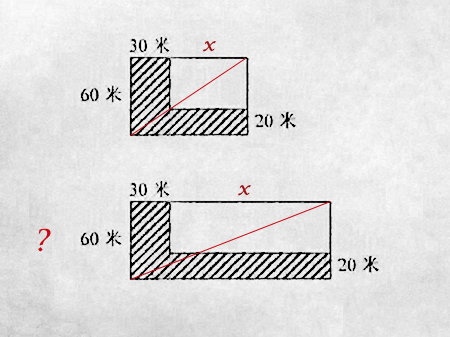x/(30+x) = (60-20)/60

x * 60 = 40 * (30+x)

60x = 1200 + 40x

x = 60

---但是无法确认等比关系。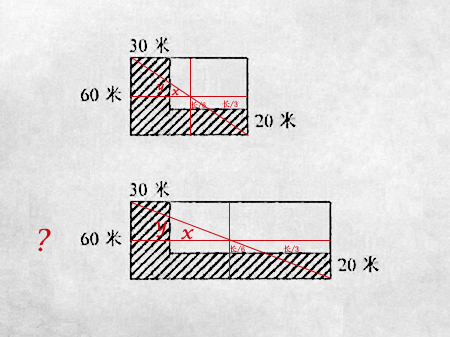x/(x+长/2)=y/(y+30)；

y/30=x/(x+30)；

x/(x+长/6)=y/(y+10)；

---但是不知道 "长/6" 关系怎么来的。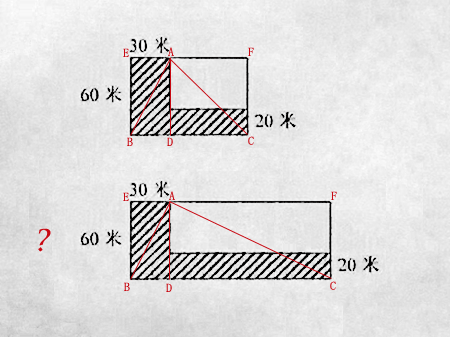3、要用到直角三角形中的射影定理（解出DC长度）

60×60=30×DC

3600=30×DC

DC=120（米）

4、阴影部分面积计算：

=EB×BC-AG×DC

=EB×（BD+DC）

=60×（30+120）-（60-20）×120

=4200（平方米）

=60×30+120×20=4200（平方米）

---但是不知道 A角 是否是直角，就用射影定理了。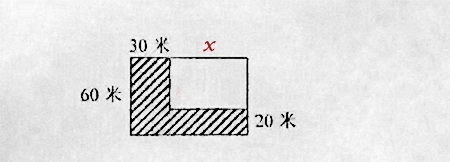---最直接粗暴的解法。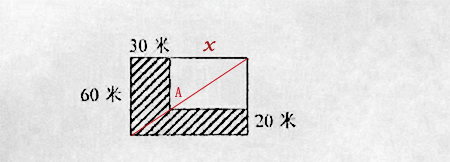---好像我读书时候也这么干过，但老师有同情心给了2分。

---说到我心坎里了。

......